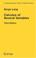### Mathematics 2

#### Course Description

Vectors. Analytic geometry in space. Differential calculus of several variables. Series. Power series. Taylor series. Ordinary differential equations.

#### General Competencies

Student is supposed to achieve a good level of understanding of theoretic concepts and principles in the topics of series, power series, Taylor series, analytic geometry in space and differential calculus of several real variables. Student will be able to select and apply corresponding methods and techniques of calculations from topics mentioned above. Student will synthesize all above competences and use it in the solving of ordinary differential equations(ODE's). Student will be able to design the differential equation, as a mathematical model, related to simple examples of the engineering practice.

#### Learning Outcomes

1. Understand the notion of convergence of series of numbers and apply the basic criteria for convergence of series of numbers.
2. Determine the area of convergence of power series.
3. Develop the function in a Taylor power series.
4. Apply tecniques and procedures on problems related to lines and planes in the space.
5. Understand basic techniques in calculus of several variables.
6. Apply calculus of several variables on finding local and global extremas of differentiable functions of several variables.
7. Relate techiques of Mathematics 2 and Mathematics 1 and use them to solve basic types of ordinary differential equations
8. Create a mathematical model, based on the differential equation, related to electircal engineering

#### Forms of Teaching

Lectures

Lectures which contain a large number of examples and problems

Exams

Mid-term and final exam during the lecture-free weeks.

Exercises

More examples for students which need more practice.

Experimental Exercises

Individually, implement by best students from higher years of study.

Consultations

At least once a week.

Continuous Assessment Exam
Quizzes 0 % 20 % 0 % 20 %
Mid Term Exam: Written 0 % 40 % 0 %
Final Exam: Written 0 % 40 %
Exam: Written 0 % 80 %
##### Comment:

The scores achieved on short tests will be transferred to the score of the exam only in the case when it is in the interest of the student.

#### Week by Week Schedule

1. VECTORS. Operations with vectors and linear combination of vectors. Coordinate system and canonical basis. Scalar product of two vectors and the angle between them. Vector product, scalar triple product and vector triple product of vectors. Liner independence of vectors and basis decomposition of vectors.
2. LINE AND THE PLANE. Plane in space and equation of the plane. Line in space and equation of the line. Mutual relationship between the line and the plane.
3. FUNCTION OF SEVERAL VARIABLES (AN INTRODUCTION) Euclidean space R^n. The notion of the graph of the function in several variables. An overview of some surfaces in Euclidean space. The equation of the curve in space. Level curves and level surfaces.
4. CALCULUS OF SEVERAL VARIABLES. (1st PART) Limit and continuity of functions in several variables. Partial derivatives. The notion of the gradient and differentiable function in several variables. Higher order derivatives and Schwartz theorem. Approximations of functions using the first differential.
5. CALCULUS OF SEVERAL VARIABLES (2nd PART) Derivatives of composite functions and chain rule. Derivatives of vector functions and tangent line on the space curve. Tangent plane. Integrals depending on the parameter.
6. APPLIED CALCULUS OF SEVERAL VARIABLES (1st PART) Directional derivative Mean value theorem Derivation of implicit function High-order differentials. 2nd Differential and quadratic forms Taylor's formula for functions of two variables
7. APPLIED CALCULUS OF SEVERAL VARIABLES (2nd PART) Extreme values of linear functions Local extreme of function of several variables Extreme of function subject to constraints. Lagrange multiplier
8. MIDTERM EXAM
9. SERIES Definition of series and convergence of series. Criteria for convergence of series. Absolute and conditional convergence. Product of series.
10. POWER SERIES AND TAYLOR SERIES Power series, area of convergence and radius of convergence. Taylor series of elementary functions. Derivatives and integrals of power series. Convergence of sequence of functions. Series of functions.
11. FIRST-ORDER DIFFERENTIAL EQUATION (1st PART) The notion of differential equation. The field of directions. Equations with separated variables. Homogeneous equations. Ortogonal trajectories Linear differential equations of the first order and applications.
12. FIRST-ORDER DIFFERENTIAL EQUATION (2nd PART) Exact differential equations. Solving using parameters. Existence and uniquness of the solution. Singular solutions of differential equations of the first order.
13. HIGH-ORDER DIFFERENTIAL EQUATION Solving the differential equation by decreasing the order. Existence and uniquness of the solution. Linear differential equation of the second order. Linear differential equation of the second order with constant coefficients. Applications of the linear differential equation.
14. LINEAR HIGH-ORDER DIFFERENTIAL EQUATION Vector subspaces and linear operators. Differential operators. Higher order homogeneous differential equations. Finding the particular solution. Linear differential equation of higher order with constant coefficients. Euler equation.
15. FINAL EXAMS

#### Study Programmes

[FER2-HR] Electrical Engineering and Information Technology and Computing - study
(2. semester)

#### Literature

N. Elezović (1999.), Linearna algebra, Element
P. Javor (1999.), Matematička analiza 2, Element,
B. P. Demidovič (1998.), Zbirka zadataka iz matematičke analize za tehničke fakultete, Tehnička knjigaSerge Lang (1987.), Calculus of Several Variables, Third Edition, Springer
M. Pašić (2004.), Matematička analiza 2, Merkur ABD

#### General

ID 86476
Summer semester
7 ECTS
L0 English Level
L2 e-Learning
90 Lectures
0 Seminar
15 Exercises
0 Laboratory exercises
0 Project laboratory